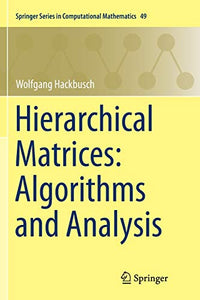# Hierarchical Matrices: Algorithms and Analysis

Wolfgang Hackbusch

Format: Print Book

ISBN: 9783662568941

• SGD 171.59
Unit price per
• Save SGD 19.07
Tax included.

Will not ship until

This self-contained monograph presents matrix algorithms and their analysis. The new technique enables not only the solution of linear systems but also the approximation of matrix functions, e.g., the matrix exponential. Other applications include the solution of matrix equations, e.g., the Lyapunov or Riccati equation. The required mathematical background can be found in the appendix.

The numerical treatment of fully populated large-scale matrices is usually rather costly. However, the technique of hierarchical matrices makes it possible to store matrices and to perform matrix operations approximately with almost linear cost and a controllable degree of approximation error. For important classes of matrices, the computational cost increases only logarithmically with the approximation error. The operations provided include the matrix inversion and LU decomposition.

Since large-scale linear algebra problems are standard in scientific computing, the subject of hierarchical matrices is of interest to scientists in computational mathematics, physics, chemistry and engineering.

Publication Year: 2015
Imprint: Springer Berlin Heidelberg
Format: P
Weight (Gram): 819